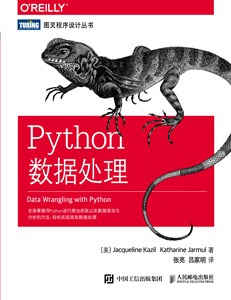2014-04-16 09:28:04 oMuYeJingFeng1 阅读数 8511
• ###### Python数据分析实战-Pandas

深度学习、机器学习和数据分析必须用pandas。pandas是在python直接流行的数据处理框架。可以说，如果不会使用pandas，就谈不上会用python做数据分析。本课程会使用奥林匹克一个真实的数据作为实验数据，从初级到各种pandas的常用操作，到常用的数据可视化，让你在短的时间内掌握好pandas，轻松愉快的玩转数据分析。

2340 人正在学习 去看看 阿勒拉哈

clientid      shopingid      num    date

1111000   3873             2          4月5日

```clientinfo = []
shopinginfo = {}
month={}
day={}
shopidflag = 0
clientstartflag = 0
total={}
tmpclientid=''
output= open('f:/a.txt','a')
with open('f:/s.txt','r') as data_file:
for lineinfo in data_file:
lineinfo = lineinfo.split()
clientid = lineinfo
shopingid = lineinfo
num=[]
num.append(lineinfo)
data = lineinfo
data = data[:-1]
data = data.split('月')
monthvar=[]
monthvar.append(data)
dayvar=[]
dayvar.append(data)

if clientid in clientinfo and shopingid in shopinginfo and int(data)>=6:
shopinginfo[shopingid].append(lineinfo)
month[shopingid].append(data)
day[shopingid].append(data)
elif clientid in clientinfo and shopingid not in shopinginfo and int(data)>=6:
shopinginfo[shopingid]=num
month[shopingid]= monthvar
day[shopingid] = dayvar
elif clientid not in clientinfo :
#if clientstartflag  == 1:
clientflag = 0
for (k, v) in shopinginfo.items():
total={}
vote=0
for  i  in v:
if  i  in total:
total[i]+=1
else:
total[i]=1
for var in total:
if var == '0':
vote += total[var]
elif var == '1':
vote = 0
break
elif var == '2':
vote += total[var]*2
else:
vote += total[var]*3
if vote >= 3:
if clientflag == 0:
output.write(tmpclientid+'\t')
clientflag =1
if clientflag == 1:
shopinginfo={}
month ={}
day ={}
clientinfo=[]
tmpclientid=clientid
clientinfo.append(clientid)
shopinginfo[shopingid]=num
month[shopingid] = monthvar
day[shopingid] = dayvar
for (k, v) in shopinginfo.items():
for  i  in v:
if  i  in total:
total[i]+=1
else:
total[i]=1
total={}
vote=0
for  i  in v:
if  i  in total:
total[i]+=1
else:
total[i]=1
for var in total:
if var == '0':
vote += total[var]
elif var == '1':
vote = 0
break
elif var == '2':
vote += total[var]*2
else:
vote += total[var]*3
if vote >= 3:
if clientflag == 0:
clientflag =1
if clientflag == 1:
output.write(tmpclientid+'\t')
data_file.close()
output.close()```

python 如何处理大数据 相关内容

2019-05-03 21:29:45 xuexijiaqq3533076323 阅读数 139
• ###### Python数据分析实战-Pandas

深度学习、机器学习和数据分析必须用pandas。pandas是在python直接流行的数据处理框架。可以说，如果不会使用pandas，就谈不上会用python做数据分析。本课程会使用奥林匹克一个真实的数据作为实验数据，从初级到各种pandas的常用操作，到常用的数据可视化，让你在短的时间内掌握好pandas，轻松愉快的玩转数据分析。

2340 人正在学习 去看看 阿勒拉哈`Python学习群：692649595，有大牛答疑，有资源共享！是一个非常不错的交流基地！欢迎喜欢Python的小伙伴！`

01、大型文件的读取效率

with open(filename,"rb") as f:
for fLine in f:
pass

02、文本处理效率问题

1.列表处理
def fun(x):尽量选择集合、字典数据类型，千万不要选择列表，列表的查询速度会超级慢，同样的，在已经使用集合或字典的情况下，不要再转化成列表进行操作，比如：

values_count = 0
# 不要用这种的
if values in dict.values():
values_count += 1
# 尽量用这种的
if keys,values in dict:
values_count += 1

2. 对于文件属性

return '(' + str(x) + ', 1)'
list(map(fun,[1,2,3]))

3. 对于字典

>>> d = {'a':1,'b':2}
>>> for i in d.items() :
.... print i
('a',1)
('b',2)
>>> for k,v in d.iteritems() :
... print k,v
('a',1)
('b',2)

`Python学习群：692649595，有大牛答疑，有资源共享！是一个非常不错的交流基地！欢迎喜欢Python的小伙伴！`

python 如何处理大数据 相关内容

2019-05-27 19:13:25 Charein 阅读数 887
• ###### Python数据分析实战-Pandas

深度学习、机器学习和数据分析必须用pandas。pandas是在python直接流行的数据处理框架。可以说，如果不会使用pandas，就谈不上会用python做数据分析。本课程会使用奥林匹克一个真实的数据作为实验数据，从初级到各种pandas的常用操作，到常用的数据可视化，让你在短的时间内掌握好pandas，轻松愉快的玩转数据分析。

2340 人正在学习 去看看 阿勒拉哈### 本书特色

• 快速了解Python基本语法、数据类型和语言概念
• 概述数据的获取与存储方式
• 清洗数据并格式化，以消除数据集中的重复值与错误
• 学习何时对数据进行标准化，何时对数据清理进行测试并将其脚本化
• 使用Scrapy写网络爬虫
• 利用新的Python库和技术对数据集进行探索与分析
• 使用Python解决方案将整个数据处理过程自动化

“如果你一直感觉电子表格（甚至关系型数据库）无法回答你想要提出的问题，或者除这些工具之外你准备进一步学习，那么这本书非常适合你。我一直在等待这本书的出现。”

——Derek Willis，ProPublica新闻应用开发者，OpenElections联合创始人

“所有新手数据科学家、数据工程师或其他技术方面的数据专家都应该读一读这本实践指南。数据处理领域正需要这样一本书，真希望我第一次开始用Python处理数据时就能有它指导。”

——Tyrone Grandison博士，Proficiency Labs Intl. CEO

### 目录

O’Reilly Media, Inc.介绍

### 下载

https://pan.baidu.com/s/1B2H_CxsXfnjRouxU6bCTRw

python 如何处理大数据 相关内容

2018-08-23 18:41:45 a40850273 阅读数 243
• ###### Python数据分析实战-Pandas

深度学习、机器学习和数据分析必须用pandas。pandas是在python直接流行的数据处理框架。可以说，如果不会使用pandas，就谈不上会用python做数据分析。本课程会使用奥林匹克一个真实的数据作为实验数据，从初级到各种pandas的常用操作，到常用的数据可视化，让你在短的时间内掌握好pandas，轻松愉快的玩转数据分析。

2340 人正在学习 去看看 阿勒拉哈

# Python 数据处理

### 数据的读取与保存

``````import pandas as pd

### DataFrame 按列差分

``d_vel = abs(data.diff()).fillna(method='bfill')``

### 数据插值

interp1d 函数通过传入的横纵坐标数据拟合并返回一个函数。这个函数可以接受一个序列作为输入，并返回相应大小的拟合插值。

``````from scipy.interpolate import interp1d

function = interp1d(range(N), data[i], kind='quadratic') # interp1d(x, y) 一维插值
fdata = function(np.linspace(0, N-1, INSERT_N * N))``````

``````from scipy import interp, arange, exp

x = arange(0, 10)
y = exp(-x/3.0)

interp([9, 10], x, y)
# array([0.04978707, 0.04978707])
``````

### 中值滤波

``````import scipy.signal as signal

x_med = signal.medfilt(x, 3) # 核为3``````

### 分位数计算

percentile 函数根据传入的数据，给出对应 25%、50%和75%的分位数

``````import numpy as np

per = np.percentile(x, [25, 50, 75])``````

### 离散信号的极值点

``````import scipy.signal as signal

extreme_geater = signal.argrelextrema(data, np.greater) # 给出的是位置标号
extreme_less = signal.argrelextrema(data, np.less)``````

### 绘图使用两个独立的坐标轴

``````import matplotlib.pyplot as plt

plt.figure()
ax1 = plt.subplot(111)
ax1.plot(range(N), f_data)
ax2 = ax1.twinx() # 独立两个y轴，x轴用twiny()
ax2.plot(range(N), data)``````

python 如何处理大数据 相关内容

2019-05-27 08:51:32 sunyaowu315 阅读数 196
• ###### Python数据分析实战-Pandas

深度学习、机器学习和数据分析必须用pandas。pandas是在python直接流行的数据处理框架。可以说，如果不会使用pandas，就谈不上会用python做数据分析。本课程会使用奥林匹克一个真实的数据作为实验数据，从初级到各种pandas的常用操作，到常用的数据可视化，让你在短的时间内掌握好pandas，轻松愉快的玩转数据分析。

2340 人正在学习 去看看 阿勒拉哈
``````def missing_delete_var(df, threshold=None):
"""
df:数据集
threshold:缺失率删除的阈值

return :删除缺失后的数据集
"""
df2 = df.copy()
missing_df = missing_cal(df)
missing_col_num = missing_df[missing_df.missing_pct >= threshold].shape
missing_col = list(missing_df[missing_df.missing_pct >= threshold].col)
df2 = df2.drop(missing_col, axis=1)
return df2

def missing_delete_user(df, threshold=None):
"""
df:数据集
threshold:缺失个数删除的阈值

return :删除缺失后的数据集
"""
df2 = df.copy()
missing_series = df.isnull().sum(axis=1)
missing_list = list(missing_series)
missing_index_list = []
for i, j in enumerate(missing_list):
if j >= threshold:
missing_index_list.append(i)
df2 = df2[~(df2.index.isin(missing_index_list))]
return df2

def const_delete(df, col_list, threshold=None):
"""
df:数据集
col_list:变量list集合
threshold:同值化处理的阈值

return :处理后的数据集
"""
df2 = df.copy()
const_col = []
for col in col_list:
const_pct = df2[col].value_counts().iloc / df2[df2[col].notnull()].shape
if const_pct >= threshold:
const_col.append(col)
df2 = df2.drop(const_col, axis=1)
return df2

def missing_cal(df):
"""
df :数据集

return：每个变量的缺失率
"""
missing_series = df.isnull().sum() / df.shape
missing_df = pd.DataFrame(missing_series).reset_index()
missing_df = missing_df.rename(columns={'index': 'col',
0: 'missing_pct'})
missing_df = missing_df.sort_values('missing_pct', ascending=False).reset_index(drop=True)
return missing_df

def data_processing(df, target):
"""
df:包含了label和特征的宽表

return:
df :清洗后的数据集
"""
# 特征缺失处理
df = missing_delete_var(df, threshold=0.8)
# 样本缺失处理
df = missing_delete_user(df, threshold=int(df.shape * 0.8))
col_list = [x for x in df.columns if x != target]
# 常变量处理
df = const_delete(df, col_list, threshold=0.9)
desc = df.describe().T
# 剔除方差为0的特征
std_0_col = list(desc[desc['std'] == 0].index)
if len(std_0_col) > 0:
df = df.drop(std_0_col, axis=1)
df.reset_index(drop=True, inplace=True)

# 缺失值计算和填充
miss_df = missing_cal(df)
cate_col = list(df.select_dtypes(include=['O']).columns)
num_col = [x for x in list(df.select_dtypes(include=['int64', 'float64']).columns) if x != 'label']

# 分类型特征填充
cate_miss_col1 = [x for x in list(miss_df[miss_df.missing_pct > 0.05]['col']) if x in cate_col]
cate_miss_col2 = [x for x in list(miss_df[miss_df.missing_pct <= 0.05]['col']) if x in cate_col]
num_miss_col1 = [x for x in list(miss_df[miss_df.missing_pct > 0.05]['col']) if x in num_col]
num_miss_col2 = [x for x in list(miss_df[miss_df.missing_pct <= 0.05]['col']) if x in num_col]
for col in cate_miss_col1:
df[col] = df[col].fillna('未知')
for col in cate_miss_col2:
df[col] = df[col].fillna(df[col].mode())
for col in num_miss_col1:
df[col] = df[col].fillna(-999)
for col in num_miss_col2:
df[col] = df[col].fillna(df[col].median())

return df, miss_df

``````

对数据分析、机器学习、数据科学、金融风控等感兴趣的小伙伴，需要数据集、代码、行业报告等各类学习资料，可添加微信：wu805686220（记得要备注喔！），也可关注微信公众号：风控圏子（别打错字，是圏子，不是圈子，算了直接复制吧！）• 机器学习风控讨论群（微信群）
• 反欺诈讨论群（微信群）
• python学习交流群（微信群）
• 研习社资料（qq群：102755159）（干货、资料、项目、代码、报告、课件）

python 如何处理大数据 相关内容